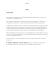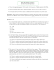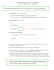# 2089-1-11-tute132014-10-29 06:38178 KB

## Transcription

2089-1-11-tute132014-10-29 06:38178 KB
```MATH 2089 - Statistics
Tutorial class Week 13 : Regression Analysis and ANOVA
Instructions
The exercises that will be gone over during the tutorial class will be chosen from those printed here.
Note that not every topic in the course can be covered by the tutorial exercises. You are expected
to take an active part in the learning process, by working by yourself on most of the topics.
In particular, you are expected to attempt these tutorial questions beforehand. At the tutorial,
the tutor will go through the answers to some of the questions, directing explanation to areas where students
indicate they have difficulty.
Solutions to these exercises will be available from the course web page later.
Exercise 1
In a certain chemical process the reaction time Y (in hours) is known to be related to the temperature
X (in °F) in the chamber in which the reaction time takes place according to the simple linear equation
Y = 5.00 − 0.01X + ε,
where ε is a random disturbance normally distributed with standard deviation σ = 0.075.
a) What is the true average change in reaction time associated with a 1 °F increase in temperature?
A 10 °F increase in temperature?
b) What is the true average reaction time when the temperature is 200 °F? When the temperature is
250 °F?
c) What is P(2.4 < Y < 2.6) when X = 250?
d) If an investigator makes five independent experimental runs for a temperature of 250 °F what is
the probability that all five observed reaction times are between 2.4 and 2.6 hours?
Exercise 2
A regression of Y = calcium content (g/l) on X = dissolved material (mg/cm2 ) was reported in a
1997 article in the Magazine of Concrete Research. The equation of the least squares regression line
was determined :
yˆ(x) = 3.678 + 0.144 × x with r2 = 0.860 and n = 23.
a) Interpret the estimated slope 0.144 and the coefficient of determination 0.860.
b) What would be a point estimate of the true average calcium content when the amount of dissolved
material is 50 mg/cm2 ?
c) The value of the total sum of squares was sst = 320.398. Calculate an estimate of the error standard
deviation in the simple linear regression model.
Exercise 3
Mist (airborne droplets or aerosols) is generated when metal-removing fluids are used in machining
operations to cool and lubricate the tool and work-piece. Mist generation is a concern of the OSHA (Occupational Safety and Health Administration), which has recently lowered substantially the workplace
standard. In a 2002 article in the Lubrication Engineering journal the following data was provided on :
X = fluid flow velocity for a 5% soluble oil (cm/sec)
Y = the extent of mist droplets having diameters smaller than 10 mm (mg/m3 )
177 189 354 362 442 965
xi 89
yi 0.40 0.60 0.48 0.66 0.61 0.69 0.99
1
Normal Probability Plot of the Residuals
(response is extentmist)
99
Percent
95
90
80
70
60
50
40
30
20
10
5
1
-0.10
-0.05
0.00
0.05
Residual
0.10
Fitted Line Plot
extentmist = 0.4041 + 0.000621 fluidvelocity
1.2
Regression
95% CI
95% PI
extentmist
1.0
S
R-Sq
0.8
0.6
0.4
0.2
0
200
400
600
fluidvelocity
4
800
1000
0.0540453
93.1%
91.7%
2
Various regression output and graphics are included for this question. Use these in your answers to
the following questions :
a) The investigators performed a simple linear regression analysis to relate the two variables. Does a
scatter plot of the data support this strategy?
b) What proportion of observed variation in mist can be attributed to the linear relationship between
velocity and mist?
c) The last observation has high leverage in fitting the regression. Explain this concept and comment
on the likely impact that removing this point from the fit would have on the proportion of observed
variation in mist that is attributable to the simple linear regression.
d) The investigators were particularly interested in the impact on mist of increasing velocity from 100
to 1000 (a factor of 10 corresponding to the difference between the smallest and largest x values in
the sample). When X increases in this way is there substantial evidence (with α = 0.05) that the
true average increase in Y is less than 0.6?
e) Estimate the true average change in mist associated with a 1 cm/sec increase in velocity and do so
in a way that conveys information about precision and reliability.
f) Comment on the normal quantile plot for residuals.
g) Provide a 95% confidence interval for the true average mist when fluid flow velocity is set at 500
cm/sec. Compare this with the graphical display.
h) Provide a 95% prediction interval for a future value of average mist that will be observed when the
fluid flow is set at 500 cm/sec. Compare this with the graphic display.
i) Explain why the interval in part h) is wider than that in part g).
j) OHSA’s draft standard requires that the mist exceed 1 mg/m3 at most 2.5% of the time. Use the
attached graph to determine the appropriate level of fluid velocity to meet this requirement.
1
0.9
Extent mist
0.8
0.7
0.6
0.5
0.4
0
100
200
300
400
500
600
Fluid velocity
700
800
Regression Analysis: extentmist versus fluid velocity
The regression equation is
extentmist = 0.404 + 0.000621 fluidvelocity
Predictor
Constant
fluidvelocity
S = 0.0540453
Coef
0.40412
0.00062108
SE Coef
0.03459
0.00007579
R-Sq = 93.1%
T
11.68
8.19
P
0.000
0.000
Analysis of Variance
Source
Regression
DF
1
SS
0.19614
MS
0.19614
F
67.15
P
0.000
900
1000
3
Residual Error
Total
5
6
0.01460
0.21074
0.00292
Values of Predictors for New Observations
New
Obs
1
fluidvelocity
500
Predicted Values for New Observations
New
Obs
1
Fit
0.7147
SE Fit
0.0227
95% CI
(0.6562, 0.7731)
95% PI
(0.5639, 0.8654)
Exercise 4
An experiment was carried out to compare the flow rates of 6 different types of nozzles, types A, B,
C, D, E and F. ANOVA calculations yielded an observed F value f0 = 4.2 from 66 observations. State
H0 and Ha for the analysis of variance, and carry out the hypothesis test at level α = 0.05, giving the
range of values for the p-value which can be determined from the tables, and stating your conclusion
in plain language. What assumptions need to be made for an ANOVA to be an appropriate test? How
could you check if they are plausible assumptions?
Exercise 5
An experiment was carried out to compare the yield of 4 different crops. Random samples were
taken of size 12 from each crop. The observed msEr was 8.2 and the observed value of the test statistic
was f0 = 2.8.
a) Draw up and complete the ANOVA table.
b) Carry out the test using a significance level of α = 0.01. Include the statements of H0 and
Ha , rejection criterion, observed value of the test statistic, p-value, and your conclusion in plain
language.
Exercise 6
Having a pet may reduce the owner’s stress level. To examine this effect, researchers recruited 45
women who said they were dog lovers. The subjects were randomly assigned to three groups of fifteen
women. One group did a stressful task alone; one group with a good friend present; and one group
with their dog present. The heart rate during the task is one measure of the effect of stress. Heart
rates (beats per minute) during stress were recorded for these 45 women. Below you see the computer
ANOVA output for these data.
Analysis of Variance for Beats
Source
DF
SS
MS
Group
2
2387.7
1193.8
Error
42
3561.3
84.8
Total
44
5949.0
Level
Control
Friend
Pet
N
15
15
15
Pooled StDev =
Mean
82.524
91.325
73.483
9.208
StDev
9.242
8.341
9.970
F
14.08
P
0.000
Individual 95% CIs For Mean
Based on Pooled StDev
-----+---------+---------+---------+(-----*-----)
(-----*-----)
(-----*-----)
-----+---------+---------+---------+72.0
80.0
88.0
96.0
4
a) What are the null and alternative hypothesis being tested?
b) From the ANOVA output find the observed value of the F -statistic and the corresponding p-value.
State both degrees of freedom for the F -statistic.
d) What are the sample means for the heartrates of the three groups of women in the study?
e) There are three groups considered in this study. If you carry out t-tests to compare them two at
a time (pairwise t-tests), how many t-tests are there? Using the Bonferroni adjustment, carry out
these t-tests and state your conclusion.
Exercise 7
A university development director conducted a survey of starting salaries offered to education majors in three states. Ten offers were recorded for each state, and a partially completed ANOVA table
appears below.
Source
Treatment
Error
Total
df
SS
MS
F
A 17470016
B
E
27
D
874482.48
C 41081016
a) Complete the ANOVA table by determining the values for A, B, C, D, E.
b) Test to see if there is a significant difference in mean starting salaries between states at level
α = 0.05. Clearly state the null and alternative hypothesis both in mathematical terms and in
simple language.
End of the tutorial class for Week 13.
```

### Cool Mist Ultrasonic Humidifier ION50 C H I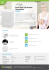### Two Devices. In One. Mist Fogger/Blower.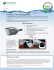### Formula 409 All Purpose Cleaner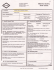### Assignment 2B-2: Regression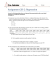### 7420 Hydraulic Oil - Steelco Industrial Lubricants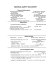### Warm/Cool Humidifier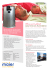### a guerrilla restaurant by Chef Gavin Baker the world`s best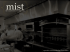### Statistics 512 Divisions 1 and 4, Spring 2014 Sample Midterm I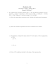### Elementary Statistics Sample Exam #3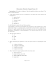### LRTrek - ActFX Algorithmic Trading